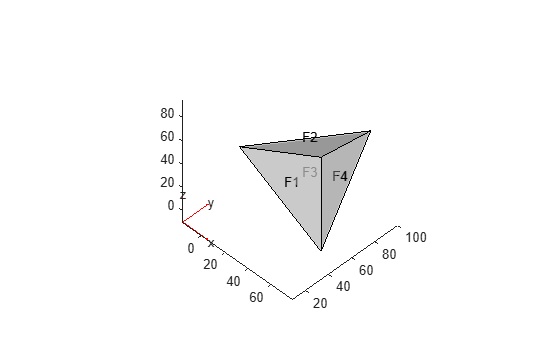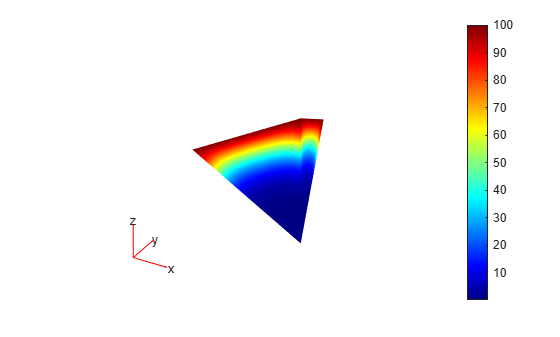# createPDEResults

Create solution object

This page describes the legacy workflow. New features might not be compatible with the legacy workflow. For the corresponding step in the recommended workflow, see `solvepde` and `solvepdeeig`.

The original (R2015b) version of `createPDEResults` had only one syntax, and created a `PDEResults` object. Beginning with R2016a, you generally do not need to use `createPDEResults`, because the `solvepde` and `solvepdeeig` functions return solution objects. Furthermore, `createPDEResults` returns an object of a newer type than `PDEResults`. If you open an existing `PDEResults` object, it is converted to a `StationaryResults` object.

If you use one of the older solvers such as `adaptmesh`, then you can use `createPDEResults` to obtain a solution object. Stationary and time-dependent solution objects have gradients available, whereas `PDEResults` did not include gradients.

## Syntax

``results = createPDEResults(model,u)``
``````results = createPDEResults(model,u,"stationary")``````
``results = createPDEResults(model,u,utimes,"time-dependent")``
``results = createPDEResults(model,eigenvectors,eigenvalues,"eigen")``

## Description

example

````results = createPDEResults(model,u)` creates a `StationaryResults` solution object from `model` and its solution `u`.This syntax is equivalent to ```results = createPDEResults(model,u,"stationary")```.```

example

````results = createPDEResults(model,u,utimes,"time-dependent")` creates a `TimeDependentResults` solution object from `model`, its solution `u`, and the times `utimes`.```

example

````results = createPDEResults(model,eigenvectors,eigenvalues,"eigen")` creates an `EigenResults` solution object from `model`, its eigenvector solution `eigenvectors`, and its eigenvalues `eigenvalues`.```

## Examples

collapse all

Create a `StationaryResults` object from the solution to an elliptic system.

Create a PDE model for a system of three equations. Import the geometry of a bracket and plot the face labels.

```model = createpde(3); importGeometry(model,"BracketWithHole.stl"); figure pdegplot(model,"FaceLabels","on") view(30,30) title("Bracket with Face Labels")``````figure pdegplot(model,"FaceLabels","on") view(-134,-32) title("Bracket with Face Labels, Rear View")```Set boundary conditions: face 3 is immobile, and there is a force in the negative `z` direction on face 6.

```applyBoundaryCondition(model,"dirichlet","Face",4,"u",[0,0,0]); applyBoundaryCondition(model,"neumann","Face",8,"g",[0,0,-1e4]);```

Set coefficients that represent the equations of linear elasticity.

```E = 200e9; nu = 0.3; c = elasticityC3D(E,nu); a = 0; f = [0;0;0];```

Create a mesh and solve the problem.

```generateMesh(model,"Hmax",1e-2); u = assempde(model,c,a,f);```

Create a `StationaryResults` object from the solution.

`results = createPDEResults(model,u)`
```results = StationaryResults with properties: NodalSolution: [14002x3 double] XGradients: [14002x3 double] YGradients: [14002x3 double] ZGradients: [14002x3 double] Mesh: [1x1 FEMesh] ```

Plot the solution for the `z`-component, which is component 3.

`pdeplot3D(model,"ColorMapData",results.NodalSolution(:,3))`Obtain a solution from a parabolic problem.

The problem models heat flow in a solid.

```model = createpde(); importGeometry(model,"Tetrahedron.stl"); pdegplot(model,"FaceLabels","on","FaceAlpha",0.5) view(45,45)```Set the temperature on face 2 to 100. Leave the other boundary conditions at their default values (insulating).

`applyBoundaryCondition(model,"dirichlet","Face",2,"u",100);`

Set the coefficients to model a parabolic problem with 0 initial temperature.

```d = 1; c = 1; a = 0; f = 0; u0 = 0;```

Create a mesh and solve the PDE for times from 0 through 200 in steps of 10.

```tlist = 0:10:200; generateMesh(model); u = parabolic(u0,tlist,model,c,a,f,d);```
```168 successful steps 0 failed attempts 324 function evaluations 1 partial derivatives 28 LU decompositions 323 solutions of linear systems ```

Create a `TimeDependentResults` object from the solution.

`results = createPDEResults(model,u,tlist,"time-dependent");`

Plot the solution on the surface of the geometry at time 100.

`pdeplot3D(model,"ColorMapData",results.NodalSolution(:,11))`Create an `EigenResults` object from the solution to an eigenvalue problem.

Create the geometry and mesh for the L-shaped membrane. Apply Dirichlet boundary conditions to all edges.

```model = createpde; geometryFromEdges(model,@lshapeg); generateMesh(model,"Hmax",0.05,"GeometricOrder","linear"); applyBoundaryCondition(model,"dirichlet", ... "Edge",1:model.Geometry.NumEdges, ... "u",0);```

Solve the eigenvalue problem for coefficients `c` = 1, `a` = 0, and `d` = 1. Obtain solutions for eigenvalues from 0 through 100.

```c = 1; a = 0; d = 1; r = [0,100]; [eigenvectors,eigenvalues] = pdeeig(model,c,a,d,r);```
``` Basis= 10, Time= 0.04, New conv eig= 0 Basis= 14, Time= 0.06, New conv eig= 0 Basis= 18, Time= 0.06, New conv eig= 1 Basis= 22, Time= 0.06, New conv eig= 3 Basis= 26, Time= 0.07, New conv eig= 3 Basis= 30, Time= 0.07, New conv eig= 5 Basis= 34, Time= 0.07, New conv eig= 5 Basis= 38, Time= 0.07, New conv eig= 7 Basis= 42, Time= 0.07, New conv eig= 9 Basis= 46, Time= 0.07, New conv eig= 11 Basis= 50, Time= 0.08, New conv eig= 11 Basis= 54, Time= 0.09, New conv eig= 14 Basis= 58, Time= 0.10, New conv eig= 14 Basis= 62, Time= 0.10, New conv eig= 16 Basis= 66, Time= 0.10, New conv eig= 17 End of sweep: Basis= 66, Time= 0.10, New conv eig= 17 Basis= 27, Time= 0.12, New conv eig= 0 Basis= 31, Time= 0.12, New conv eig= 0 Basis= 35, Time= 0.12, New conv eig= 0 Basis= 39, Time= 0.12, New conv eig= 0 Basis= 43, Time= 0.13, New conv eig= 0 End of sweep: Basis= 43, Time= 0.13, New conv eig= 0 ```

Create an `EigenResults` object from the solution.

`results = createPDEResults(model,eigenvectors,eigenvalues,"eigen")`
```results = EigenResults with properties: Eigenvectors: [1440x17 double] Eigenvalues: [17x1 double] Mesh: [1x1 FEMesh] ```

Plot the solution for mode 10.

`pdeplot(model,"XYData",results.Eigenvectors(:,10))`## Input Arguments

collapse all

PDE model, specified as a `PDEModel` object.

Example: `model = createpde`

PDE solution, specified as a vector or matrix.

Example: `u = assempde(model,c,a,f);`

Times for a PDE solution, specified as a monotone vector. These times should be the same as the `tlist` times that you specified for the solution by the `hyperbolic` or `parabolic` solvers.

Example: `utimes = 0:0.2:5;`

Eigenvector solution, specified as a matrix. Suppose

• `Np` is the number of mesh nodes

• `N` is the number of equations

• `ev` is the number of eigenvalues specified in `eigenvalues`

Then `eigenvectors` has size `Np`-by-`N`-by-`ev`. Each column of `eigenvectors` corresponds to the eigenvectors of one eigenvalue. In each column, the first `Np` elements correspond to the eigenvector of equation 1 evaluated at the mesh nodes, the next `Np` elements correspond to equation 2, and so on.

Eigenvalue solution, specified as a vector.

## Output Arguments

collapse all

PDE solution, specified as a `StationaryResults` object, a `TimeDependentResults` object, or an `EigenResults` object. Create `results` using `solvepde`, `solvepdeeig`, or `createPDEResults`.

Example: `results = solvepde(model)`

## Algorithms

The procedure for evaluating gradients at nodal locations is as follows:

1. Calculate the gradients at the Gauss points located inside each element.

2. Extrapolate the gradients at the nodal locations.

3. Average the value of the gradient from all elements that meet at the nodal point. This step is needed because of the inter-element discontinuity of gradients. The elements that connect at the same nodal point give different extrapolated values of the gradient for the point. `createPDEResults` performs area-weighted averaging for 2-D meshes and volume-weighted averaging for 3-D meshes.## Version History

Introduced in R2015b

expand all